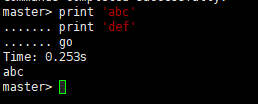``select OBJECT_DEFINITION(object_id(@object_name))``

• 通过判断 char(13),char(10) 换行回车符
• 将内容分行输出
• 并且每一行如果超过预设长度，则截断在 comma
• 再把输出结果拷贝黏贴至 excel
• 通过 excel 分列，得到完整清晰的 sql 脚本

``````Create function sql_gen  (@object_name  nvarchar(max), @return_length int)
--@object_name 为对象名，可以是存储过程，函数，视图；@return_length 为 linux shell 下每行输出的字符数量
returns @t table (content nvarchar(max) ,row int,sequence int)
--content 为根据 char(13)+char(10) 分行的内容, row 为标准应该是第几行, sequence 为长度超宽后再次截断的分行序号
as
begin

declare @object_content nvarchar(max)
declare @object_content_pre nvarchar(max)
declare @object_content_pre_reverse nvarchar(max)
declare @object_content_pre_reverse_comma int
declare @object_content_pre1 nvarchar(max)
declare @object_content_pre2 nvarchar(max)
--declare @return_length int=100.00
declare @length int
declare @position int

set @object_content=rtrim((select OBJECT_DEFINITION(object_id(@object_name))))
set @position=charindex(char(13)+char(10),@object_content)

declare @i int=1
declare @j int=1
declare @k int=1
declare @m int=1

set @i=((select len(@object_content)-len(replace(@object_content,char(13)+char(10),'')))/2)

while @j<=@i

begin
set @object_content_pre=(select top 1 left(@object_content,charindex(char(13)+char(10),@object_content)) )
set @object_content=stuff(@object_content,1,charindex(char(13)+char(10),@object_content),'')
set @object_content_pre=replace(replace(@object_content_pre,char(13),''),char(10),'')
set @length=len(@object_content_pre)
set @k=ceiling(@length/@return_length)+1

while @k<>0
begin
set @object_content_pre1=substring(@object_content_pre,1,@return_length)

set @object_content_pre_reverse=REVERSE(@object_content_pre1)
set @object_content_pre_reverse_comma=CHARINDEX(',',@object_content_pre_reverse)

set @object_content_pre1=substring(@object_content_pre,1,@return_length-@object_content_pre_reverse_comma)

set @object_content_pre=substring(@object_content_pre,@return_length+1-@object_content_pre_reverse_comma,len(@object_content_pre))

insert @t(content,row,sequence) select @object_content_pre1,@j,@m

set @length=len(@object_content_pre)
set @k=@k-1
set @m=@m+1

end

set @j=@j+1
set @m=1

end

return
end ``````

执行以下语句可获取脚本，注意 sequence 大于1的值, 代表上一行被截断，少量的手动更新脚本是可能存在的:

``select * from sql_gen('对象名称',200) order by row,sequence``

刚开始的时候，考虑过使用 存储过程中的 print 输出脚本，免得在 excel 中分列输出，但是，但是，在 mssql_cli 中 print 只能输出一行。